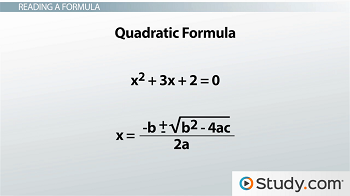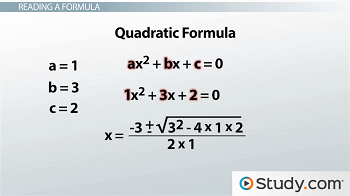# Understanding and Evaluating Math Formulas

An error occurred trying to load this video.

Try refreshing the page, or contact customer support.

Coming up next: Expressing Relationships as Algebraic Expressions

### You're on a roll. Keep up the good work!

Replay
Your next lesson will play in 10 seconds
• 0:02 Why Do We Need Math Formulas?
• 3:44 How to Choose a Formula
• 5:23 Another Example
• 6:24 Lesson Summary
Save Save

Want to watch this again later?

Timeline
Autoplay
Autoplay
Speed Speed Audio mode

#### Recommended Lessons and Courses for You

Lesson Transcript
Instructor: Yuanxin (Amy) Yang Alcocer

Amy has a master's degree in secondary education and has taught math at a public charter high school.

With all the formulas out there, how do you know which one to pick? And how do you read formulas anyways? Watch this video lesson and you will find the answers to these questions.

## Why Do We Need Math Formulas?

Why do we need to know about formulas in math? Couldn't we just get by with our basics like adding, subtracting, multiplying, and dividing? Sure, but it would take us that much longer to solve a problem. Math formulas, which are equations that show us how to work something out, tell us exactly what we need to do to solve a particular problem. If we didn't use formulas, then we would have to do all the work that led up to the formula ourselves to find our answer. For example, if we wanted to find the volume of a box, we could either use the formula for the volume of a box, V = l * w * h, or we could use our basics and spend quite a bit of time to figure out that to find the volume of a box, we need to multiply its length, width, and height. Either way we go about it, the formula finds its way in. We can either use it to help us, or we can do things the hard way. I think letting formulas help us is the way to go.

So, if we are going to use formulas, how do we read them?

Let's look at an example.

Say we want to solve a quadratic equation, which is an equation whose highest exponent is 2, such as x^2 + 3x + 2 = 0. For these types of equations, we can use the quadratic formula. The formula tells us how to solve quadratic equations. It looks like this.At first, this just looks like a bunch of letters with not much meaning. But this formula also tells us for what kinds of equations it will work. It works for equations in the form of ax^2 + bx + c = 0. Ah, we have more letters now. But this equation looks somewhat like ours. I see an x^2, an x, and a 0. The only things different here are the numbers where the letters are. What does that tell me? Why, it tells me what those letters are equal to for my problem equation. I have my a = 1, my b = 3, and my c = 2. Oh wait, would you look at that! Those are the letters in my formula. So, I can plug my numbers into the formula to find my answer. Now, I have this.Would you look at that! My formula has turned into something I can evaluate. The plus/minus symbol tells me that I will be splitting this formula up into two parts. Before I do that, though, I go ahead and evaluate as much as I can. So I multiply the 4 with the 1 and 2 to get 8. I square the 3 to get 9. I subtract the 8 from the 9 to get 1 under the square root. I multiply the 2 and the 1 in the denominator to get 2.

I'm looking even better now. What is the square root of 1? It's 1. So now, I can split my equation into two parts, one plus part and one minus part. I get x = (-3 + 1)/2 and x = (-3 - 1)/2. Evaluating both of these separately, I get x = -1 and x = -2. I got my answers!

If I didn't have the use of this formula, solving my quadratic would be that much harder and would take even more time! As we saw, reading a formula is about matching our letters to the appropriate parts of our problem and then plugging those values back into the formula. Once we have matched all our letters and plugged them in, it's just a matter of evaluating.

## How to Choose a Formula

Yes, formulas really do make our lives easier. But, how do you choose which formula to use? We do this by carefully reading our problem to find out what they are looking for. Once we know what they are looking for, then we look for the formula that deals with that question.

For example, say we have this problem:

To unlock this lesson you must be a Study.com Member.

### Register to view this lesson

Are you a student or a teacher?

#### See for yourself why 30 million people use Study.com

##### Become a Study.com member and start learning now.
Back
What teachers are saying about Study.com

### Earning College Credit

Did you know… We have over 200 college courses that prepare you to earn credit by exam that is accepted by over 1,500 colleges and universities. You can test out of the first two years of college and save thousands off your degree. Anyone can earn credit-by-exam regardless of age or education level.